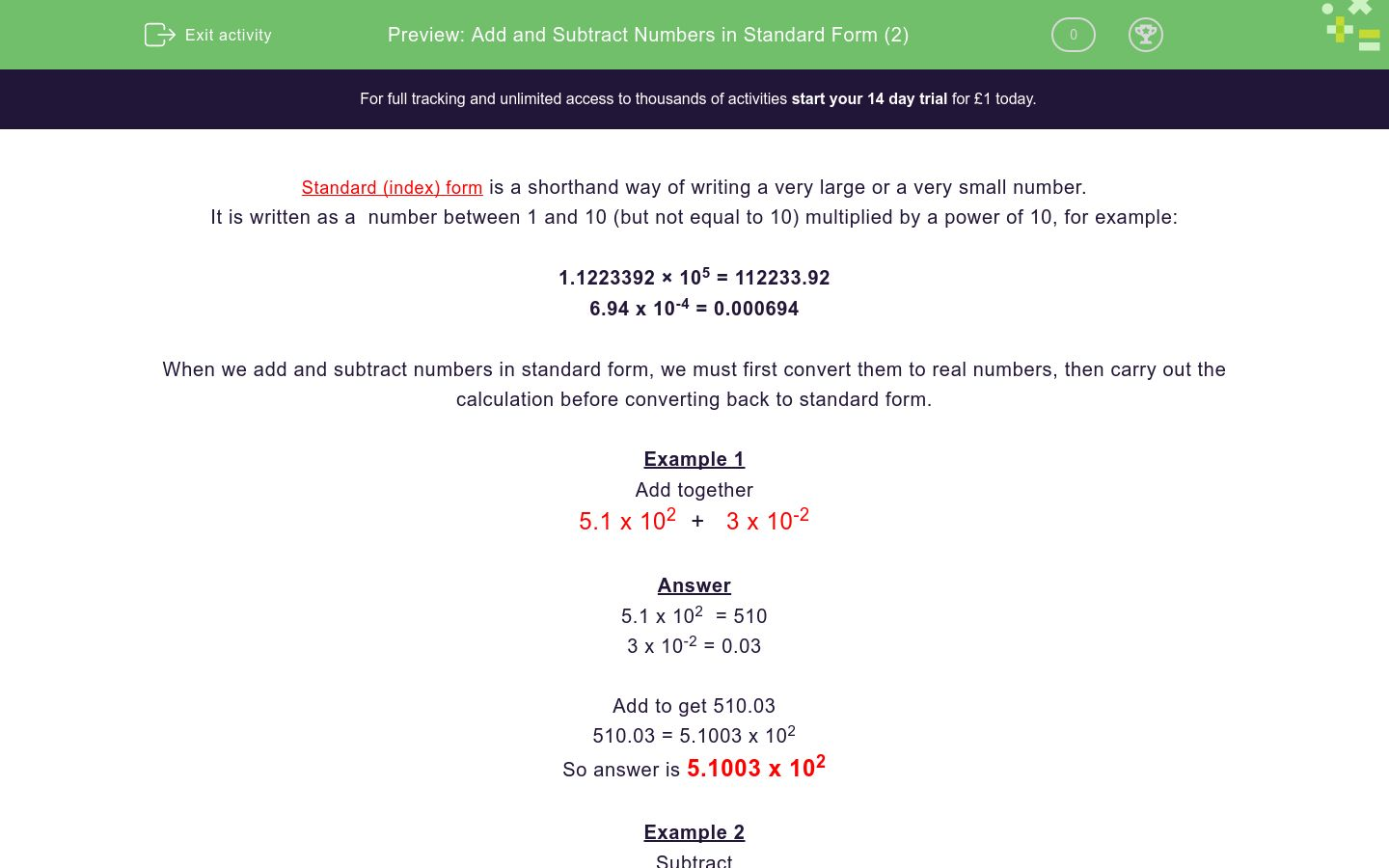# Add and Subtract Numbers in Standard Form (2)

In this worksheet, students add and subtract numbers written in standard form.Key stage:  KS 4

Curriculum topic:  Number

Difficulty level:### QUESTION 1 of 10

Standard (index) form is a shorthand way of writing a very large or a very small number.

It is written as a  number between 1 and 10 (but not equal to 10) multiplied by a power of 10, for example:

1.1223392 × 105 = 112233.92

6.94 x 10-4 = 0.000694

When we add and subtract numbers in standard form, we must first convert them to real numbers, then carry out the calculation before converting back to standard form.

Example 1

Add together

5.1 x 102  +   3 x 10-2

Answer

5.1 x 102  = 510

3 x 10-2 = 0.03

Add to get 510.03

510.03 = 5.1003 x 102

So answer is 5.1003 x 102

Example 2

Subtract

5.2 x 103  -   9 x 10-1

Answer

5.2 x 103  = 5200

9 x 10-1 = 0.9

Subtract to get 5199.1

5199.1 = 5.1991 x 103

So answer is 5.1991 x 103

Add together

4.4 x 106  +   6.6 x 104

(The answer must be in standard form)

4.6 x 106

4.466 x 106

10.1 x 1024

Add together

8 x 105  +   2 x 105

(The answer must be in standard form)

16 x 105

10 x 105

1 x 106

Subtract

3 x 102  -   4 x 10-1

(The answer must be in standard form)

3.4 x 101

2.996 x 102

2.6 x 102

Subtract

5 x 103  -   4 x 10-2

(The answer must be in standard form)

4.99996 x 103

4.9996 x 103

1 x 105

Add together

1 x 103  +   4 x 10-1

(The answer must be in standard form)

4.001 x 102

5 x 102

1.0004 x 103

Subtract

4 x 10-3  -   4 x 10-4

(The answer must be in standard form)

1 x 107

3.6 x 10-4

3.6 x 10-3

Subtract

4.2 x 10-5  -   4 x 10-7

(The answer must be in standard form)

4.16 x 10-5

2 x 1012

-4 x 107

Add together

6.3 x 10-5  +   3.24 x 10-7

(The answer must be in standard form)

9.54 x 1012

9.54x 10-7

6.3324 x 10-5

Add together

3.313 x 101  +   4.28 x 10-1

(The answer must be in standard form)

3.741 x 100

12.2 x 102

7.593 x 100

Subtract

2.5 x 10-5  -   6.35 x 10-4

(The answer must be in standard form)

-3.85 x 10-1

-6.1 x 10-4

3.85 x 10-9

• Question 1

Add together

4.4 x 106  +   6.6 x 104

(The answer must be in standard form)

CORRECT ANSWER
4.466 x 106
• Question 2

Add together

8 x 105  +   2 x 105

(The answer must be in standard form)

CORRECT ANSWER
1 x 106
• Question 3

Subtract

3 x 102  -   4 x 10-1

(The answer must be in standard form)

CORRECT ANSWER
2.996 x 102
• Question 4

Subtract

5 x 103  -   4 x 10-2

(The answer must be in standard form)

CORRECT ANSWER
4.99996 x 103
• Question 5

Add together

1 x 103  +   4 x 10-1

(The answer must be in standard form)

CORRECT ANSWER
1.0004 x 103
• Question 6

Subtract

4 x 10-3  -   4 x 10-4

(The answer must be in standard form)

CORRECT ANSWER
3.6 x 10-3
• Question 7

Subtract

4.2 x 10-5  -   4 x 10-7

(The answer must be in standard form)

CORRECT ANSWER
4.16 x 10-5
• Question 8

Add together

6.3 x 10-5  +   3.24 x 10-7

(The answer must be in standard form)

CORRECT ANSWER
6.3324 x 10-5
• Question 9

Add together

3.313 x 101  +   4.28 x 10-1

(The answer must be in standard form)

CORRECT ANSWER
3.741 x 100
• Question 10

Subtract

2.5 x 10-5  -   6.35 x 10-4

(The answer must be in standard form)

CORRECT ANSWER
-6.1 x 10-4
---- OR ----

Sign up for a £1 trial so you can track and measure your child's progress on this activity.

### What is EdPlace?

We're your National Curriculum aligned online education content provider helping each child succeed in English, maths and science from year 1 to GCSE. With an EdPlace account you’ll be able to track and measure progress, helping each child achieve their best. We build confidence and attainment by personalising each child’s learning at a level that suits them.

Start your £1 trial